﻿ Generalized Potential Operator and the Comments on Its Time EvolutionPublications are Open
Access in this journal
Article Versions
Export Article
• Normal Style
• MLA Style
• APA Style
• Chicago Style
Research Article
Open Access Peer-reviewed

### Generalized Potential Operator and the Comments on Its Time Evolution

Divyansh Mansukhani
International Journal of Physics. 2018, 6(1), 9-14. DOI: 10.12691/ijp-6-1-2
Published online: January 16, 2018

### Abstract

Potential energy inherited by a system plays a significant role in the system analysis. The potential energy operators are not generalized till date. Each system has its unique basis to explain the same. This article provides a novel approach to determine a generalized potential operator for a particle using Poisson's equation in a set basis in accordance with the corresponding characteristics manifested by the unit elements of basis. The mathematics includes the fundamental definitions of quantum physics and geometrical inference of manifolds. We shall see that the operator itself is time variant and medium dependent, which in turn depicts the evolution of the system in different mediums.

### 1. Introduction

The set of basis vectors define the coordinate system, which in turn is the tool for determining the state of any observable. A ball held at an height h over the surface of earth is proposed to have a potential energy of mgh considering the ordinate to be the altitude and abscissa to be distance parallel to Earth's surface. Mentioning a different system, a box attached to a spring and stretched by x units from the mean position is proposed to have a potential energy of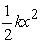in its corresponding coordinate system. Various such cases give us a non-generalized potential operators which are specific to their own corresponding systems. Thus to find spatial or time evolution of more than one systems, the operator is made to individually act on their specific systems using mapping techniques so that the ambiguous operations can be avoided. Such mapping operations occupy high computational processing considering high percentage of total mappings to be vague. Hence the objective of the article is to establish an operator in terms of position and time such that it could provide the potential energy of a particle at fed position (of any specific coordinate system) and time. Note that such an operator would signify the parameters of a specific system when acted over a function. Since it won’t compare two frames, there is no point of approaching the objective using separate relativistic and non-relativistic methods.

Let me first define a coordinate system, using which we would be achieving the objective of deriving a generalized potential operator in a mathematically comfortable manner. Let it have two basis vectors. One being the single derivative of position, i.e. velocity and other being the anti-log of mass. We see, the coordinate system is still dependent on the position and hence, we are not violating the fundamental law - "Potential Energy is the virtue of a system's position". Later in section (7), the operator is mentioned in terms of universal position coordinate system as well.

### 2. Introduction of Manifold in our Observance

Consider an particle observable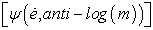in this coordinate system, e being the position. It is obvious that any observable has to be placed over the elementary units of coordinate system in order for it to be introduced under observance. Therefore if we try to define a potential manifold [V], instead of considering the particles placed over the manifold, it won’t be absurd to simply consider corresponding elementary units over which the manifold lies.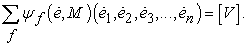Here summation over f refers to summation of different wavefunctions (later inferred as different differential strips having particular wave function which add up to make the manifold) and M is the short notation for anti-log (m). The elementary units of the coordinate system have no mass. Hence for writing an equation for the units, anti-log (m) i.e. M shall be equal to unity.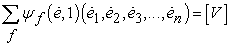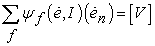(1)

The identity matrix is introduced just for the purpose of better arrangement of the equation. At this stage, we can realize that the basis anti-log (m) was introduced so as to simplify the analysis.

### 3. Variable Operator Action

When an operator acts on any vector, the vector undergoes transformations in magnitude, direction or both. Here, we are interested in an operator system which results in eigen values i.e. the eigen functions undergo changes in only magnitude. Eigen values are real numbers through which the system can be addressed. It is appropriate to state that the operation of specific operators extract a real value from the function itself which helps us to analyse that particular system.

For our case, I have considered the set of specific operators to act on the manifold [V] such that any operator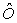and all the operators of the family till the most deviated operator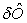is acting individually on the different strips of manifold.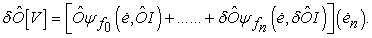(2)

We see, the operator is acting on just the identity matrix and not the basis vector. The equation can be justified using two ways:

a) The identity matrix is itself being compromised to hold the original state of basis vector. Change in such a matrix over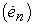results to the expansion or the contraction of the coordinate system itself.

b) An operation which extracts a real value out of the system is an operation in which the direction of the vector doesn’t change irrespective of the operator. Such an operation is feasible only if the vector resides in the direction of the basis vector. Hence, the basis vector is not needed to be operated on with a direction changing operator.

Following is the operation in terms of a generalized term: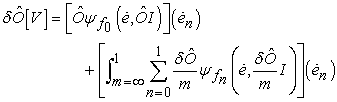(3)

where m is a variable introduced to cover the range of individual operations. Hence, the generalized term is integrated over m. On other hand, n is discrete as not all wave functions are acceptable as eigen functions. For obtaining the eigen value of such a cumulative operator system, we take m-1 from the RHS of Equation 3 as no other term is expected to give off a real value. Wave functions, by definition, are not numbers and operators are the terms by which we are primarily supposed to get rid.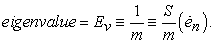(4)

Since each wave function will have a distinct eigen value,is mentioned in the above equation just to depict a general eigen value corresponding to a general eigen function. It may hence not be misinterpreted that eigen value has a direction. S is a compensation matrix extracted out of the operated matrix for it to be turned back into identity matrix.

### 4. Poisson's Equation and It's Serviceable Advantage

Poisson's Equation is one among the very famous equations of physics. It relates the potential with the charge or mass distribution density.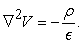In our system, the wave function is fragmented into discrete elementary wave functions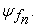Hence, applying the Poisson's Equation on each of the elementary wave functions and then summing it up shall give the averaged optimized density distribution of the whole system.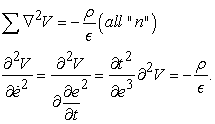(5)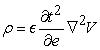(6)

where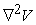is unidirectional gradient of V in the basis of position, not it's derivative. Hence, using Poisson's Equation, we have re-entered into the coordinate system containing position vectors as the basis with some fatuous improperly balanced partials. We may later see in the last section that how to meaningfully consider and perceive such mathematics.

Since density distribution is a distinct real term for each wave functions, it is not wrong to say that it would have a relation with the corresponding eigen value of the same wave function. Hence, a wave function can be addressed not only by its eigen value but also by its density distribution.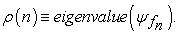Using this relation, we shall deal with a specific operation and relate the corresponding eigen value with the density distribution. Before diving into the operation, we must understand the operator-eigen value relation. Our specific operation includes operating the operator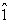on the complete unmitigated wave function. Such an operator is linear operator which gives eigen value as 1.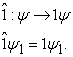Hence, the summation of eigen values of elementary wave functions must result as 1 and summation of density must result as the averaged optimized density such that the operation on the system makes no change in the system. Other way of picturing, the operatorhas to do nothing with any change because it simply multiplies with the real value 1.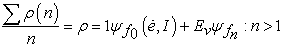(7)

Ev is mentioned in eq. (4).

### 5. Introduction of Manifolds

Till now, we have dealt with a single manifold [V]. Now, we shall one by one introduce identical manifolds and do the analysis till we would have considered d such manifolds which would have swept the whole coordinate system within them so that we could get a generalized potential operator which may not needed to be modified depending upon the different regions of coordinate system. Let us begin by introducing one manifold [W] at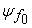i.e. let us introduce [W] such that [W] and [V] intersect at the potential strip of wave functionconsidering the unmitigated wave function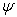to be an area of potentials. As a result, the density distribution at the strip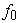will vary. Since, the manifold introduced is identical to the existing manifold, the charge or mass of which the density distribution is analyzed, will be shared equally between the two manifolds.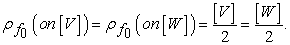Introduction of d manifolds seems to arise two methods of arranging the manifolds.

5.1. Radially Outwards from the Origin

Each manifold is arranged such that one of its edges are in contact with edges of other manifolds.

• Figure 1. 2-D description of radially outwards arrangement of manifolds

A visual 2-D description of such an arrangement is Figure 1. Note that Figure 1 is not the whole description as the manifolds are expected to occupy depth as well. Since, all the manifolds are identical, they all occupy the same depth. Thereby we can say, the “Radially Outwards from the Origin” arrangement refers to a cylindrical cavity of a finite depth made by manifolds as the cavity radius shrinks to 0. Please note that each manifold description in the figure is the average value of elementary points of manifold.

5.2. Parallel Arrangement

In such an arrangement, manifolds are arranged in juxtaposition such that one of their edges are intersecting a commonly shared manifold at individual strips. The shared manifold can be referred as the horizontal axis of Figure 2.

• Figure 2. 2-D description of parallelly arranged manifolds

Since the manifold itself is a function in coordinate system in which it is introduced, the function can be interpreted as superposition of different functions having different weights or probability amplitudes. Moreover, since the manifolds are identical and distinct, a manifold in parallel arrangement can be written only in terms of two equal magnitude functions arranged symmetrically w.r.t. the manifold.

• Figure 3. Superposition of components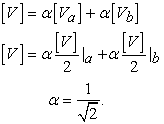The state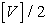must not be confused with the probability amplitude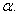It is represented in such way because volume covered by either sides of manifold are equal and hence, primarily we reprsented a random shaped manifold using an averaged plane.

• Figure 4. Average Manifold

Let us now be introduced to the concept of probability current. As mentioned earlier, since a manifold itself is a wave function, a probability density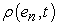exists such that: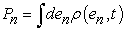Where Pn is the probability to find particle at e and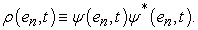Let us go ahead with mathematics: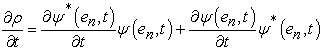(8)

1

Expressing the differential terms of eq. (8) in terms of Hamiltonian: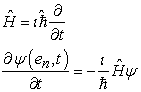(9.1)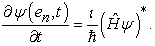(9.2)

Substituting eq. (9) in eq. (8):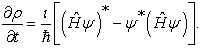(10)

We know that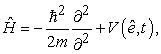deduced from many-particle Hamiltonian 2. Substituting the Hamiltonian in eq. (10), we get: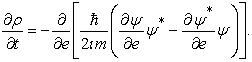Applying identity “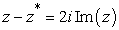3 over the above equation: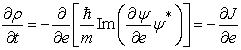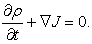(11)

Here, J is the current associated with the manifold. Eq. (10) refers to the Current Conservation Law. A peculiar way of perceiving the law is that for every change in probability density, a current is associated with the system. Making an analogy with the well-known electron current system, the current is result of motion of electrons i.e. change in the electron density in the presence a potential gradient. Hence for no current, the manifold should be such that the probability density doesn’t change with time. This is only possible if the manifold is planar.

Please note that the manifold being planar means that it occupies a set of linear levels throughout the coordinate system exactly like a paper. It should not be confused with the averaged manifold in which it could attain any set of values pertaining to the fact that the average of them should not change.

Manifolds are not something which is introduced by the mathematical operations and manipulations we do. They are the specific inputs for our system over which operations are performed. Planar manifolds are obviously the inputs for our system but not the only ones. Randomly valued envelope like manifolds are not restricted to the system. For such manifolds in parallel arrangement, although the current would be flowing individually in each of their components, the net current would be equal to 0 because the component manifolds would be equal in magnitude and equally displaced from the actual manifolds (refer Figure 3). We may say that the parallel arrangement of the manifolds welcomes only specific manifolds and thereby is not suitable for our proceedings. Hence, we shall discard it for our further analysis. Let us see what happens when a manifold is represented in two equal components in the radially outwards arrangement.

• Figure 5. Superposition – Radially Outwards from Origin

Since the components are not parallel, a net current is always present in the system making it agreeable to any unknown manifold introduced. A special case of such representation is Figure 6. The two components individually yield the current J in same direction making the net current to be maximized as 2J.

• Figure 6. Radially Outwards from Origin – Special Case

This is a case in which the current flows orthogonally from the manifold. Such directional flow of current necessitates the existence of other adjacent manifolds in the system. The radial arrangement is in accordance with what we need and hence we shall proceed taking the manifolds in such an arrangement.

All the manifolds intersect the manifold [V] at one strip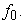Let there be d manifolds introduced. Therefore,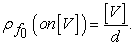(12)

### 6. Final Step – Operator Derivation

We are almost done. Eq. (6) gives a relation between the distribution density and the potential. A specific distribution density such as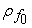will relate the potential of specific particles of the manifold which are on the particular strip; in this case,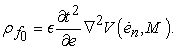Substituting it in eq.12,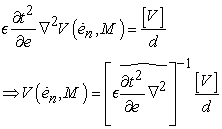(13)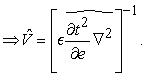(14)

Eq. (14) is the potential operator which when operated on a manifold will provide us with the potential energy associated with the particle or group of particles. The presence of d , unbalanced differencial operators, time terms, unsolved nabla squared and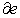and the need for applying it over a manifold may seem to add vagueness and ambiguity in understanding the meaning of the equation. We shall deal with all the necessary points in the next section.

• The coordinate system used to derive the operator has first derivative of position as one of its basis. To analyse the operator expression in position realm, we do the following operation: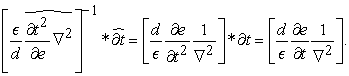We see that the position and time differential operators are balanced as the numerator and the denominator have same degree.

• Let us now do the analysis of units.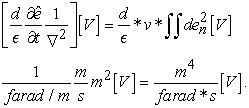We see that LHS and RHS are dimensionally not agreeing. Hence, we ought to add a dimensional proportionality constant.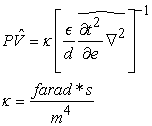Note that that operator mentioned here is in the position realm.

• The operator can also be related to Field (E) using the universal relation: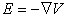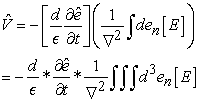[E] is the field function at a particular position. Its triple integral refers to the field enclosed in a volume. This volume may be taken as the whole coordinate system only if a constant field is applied throughout, which is not a compulsory happening. We can take advantage by creating a single field system with boundary approximations as per our requirements.

Mentioning a different perspective, a field is always associated with a manifold. Since, manifold is set of points at different continuous potential levels and potentials and fields have essentially the similar root of origin, fields to be associated with manifolds were primarily intuited.

Considering the field magnitude to be an input to the system, the operator is not supposed to act on any manifold unlike the previous representations. The only input to the expression is the position e.

• Time Evolution of the System – The fact that a time dependent term exists in the expression of the potential operator signifies that neither the operator nor the potential energy is time variant. It simply suggests that the operator is suitable to give the potential energy of particle having motion. One domain of finding its advantage is Quantum Physics where in particle showing dominant wave characteristics travel in different states simultaneously.

If inferred in terms of velocity, the operator sufficiently claims to provide the potential energy of a moving particle, nowhere mentioned that it takes the velocity to determine the same. The velocity term mentioned in the equation merely denotes the evolution of position e with time.

A time evolution operator mentioned in 3 is derived considering time independent Hamiltonian with an exponentially time evolving function. An analogy can be established showing how the operation evolves with time despite of the major term (Hamiltonian in time evolution operator and position in our case) being time independent.

It won’t be appropriate to further describe this apparently inherited factor due to its deepness and elegancy which I must not either underestimate or overestimate it.

• We see that the operator is dependent on a medium dependent characteristic.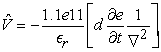Where r is the relative permittivity of the medium. Hence the operator is in accordance with the Poisson’s Equation which also shows such dependency.

• Section (6) was ended with mentioning the vagueness of the operator due to the necessity of it to be operated on a manifold [V] in order to get the potential energy. It is hard to put a meaning in words after the mathematics itself shows it. Let me still try to establish an analogy with simple classical system – a ball at altitude h. It is clear that e can be substituded as (h,x). The manifold [V] can be refered as the ball of which the study is being done. It refers to all the levels/states in which ball having altitude h could exist. In the particular classical system, there seems only one such state – same as e. Whereas if we dive into quantum scales, we’ll find that particles exist in different states and hence their will be many magnitudes of [V] for same e.

• Following is the least significant comment over a plainly expectable existance. The method using which we arrived at the operator defines the degree of operations done on manifolds. We can not simply add an integral and then say that we potential energy can be determined. The units included in the derivation are unique and optimumly enough. If, by applying more operators we get the same result, then it would signify the resemblance of various differential strips of the manifold.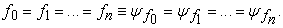This refers to a planar manifold.

### Acknowledgements

I would like to thank Prof Allan Adams, Department of Physics, MIT for contributing in 8.04 lecture series for OCW. I would also like to thank Caltech for making Feynman Lecture Series open to public. I got inspired particularly by lecture 20 – Operators. It provided a unique perspective of inferring operators at a fundamental level. http://www.feynmanlectures.caltech.edu/III_20.html.

### References

  Barton Zwiebach, “Lecture Notes: Lecture 6: 3. The Probability Current”, Feb 23-2016, MIT OCW 8.04, https://ocw.mit.edu/courses/physics/8-04-quantum-physics-i-spring-2016/lecture-notes/MIT8_04S16_LecNotes6.pdf. In article View Article  W.D. Curtis and F.R. Miller, “Differential Manifolds and Theoretical Physics: Definition 2.7” , 1985, Academic Press. [E-book]. In article View Article  “Complex Number Arithmetic (Notes): Conjugate Complex Numbers”, https://trans4mind.com/personal_development/mathematics/polynomials/ComplexNumberArithmetic.htm# mozTocId571307. In article View ArticleThis work is licensed under a Creative Commons Attribution 4.0 International License. To view a copy of this license, visit http://creativecommons.org/licenses/by/4.0/